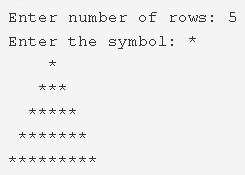# C Pyramid Star Pattern Program – Pattern Programs | C

C Program to print Pyramid Star Pattern – In this article, we will detail in on all the ways to print a Pyramid Star Pattern in C programming.

Suitable examples and sample programs have also been added so that you can understand the whole thing very clearly. The compiler has also been added with which you can execute it yourself.

The ways used in this piece are as follows:

• Using For Loop
• Using While Loop
• Using Do-While Loop

As we all know, a pyramid is a three-dimensional structure in the field of geometry. It has a square base which tapers off to a single point giving it the height or the third dimension.

A pyramid is a very popular monument-type in our world. Quite a few mausoleums, temples, tombs etc. have been built like a Pyramid.As you can see in the image uploaded above, firstly, you need to enter the number of rows.

The number of rows for this pattern is 5.

Then, choose the symbol with which you want to print your Pyramid pattern with.

The ‘*’ symbol is chosen accordingly.

So, a pyramid of ‘*’ symbol with 5 rows is printed successfully.

Hence, the numerous ways to print the same in C programming are as follows:

## Using For Loop

1. Read the row number which represents the height of the pyramid and stores that value into the variable n.

2) Using ‘scanf(“%c”,&ch)’ statement read the entered symbol and stores that symbol into the variable ‘ch’.

3) To iterate through rows run the outer for loop with the structure for(i=1;i<=n;i++),

4) The 1st inner for loop iterates through columns  with the structure for(j=0;j<n-i;j++).

a) Prints space. Each row contains (total no.of rows-row number) of spaces.

5) The 2nd inner for loop iterates through columns  with the structure for(j=0;j<(i*2)-1;j++).

a) Prints ‘*’.Each row contains (2*row number-1)of stars.

6) After each row cursor will move to the next line.

7) In the given example n=5, the symbol is’*’.So the height of the pyramid is 5.

The 1st for loop iterates from i=1 to 5.

At 1st row 2nd for loop prints 5-1 spaces,3rd for loop prints 2-1 stars, cursor comes to next line. In 2nd row 2nd for loop prints 5-2 spaces,3rd loop prints 4-1 stars, cursor comes to next line.

In 3rd row 2nd for loop prints 5-3 spaces, 3rd loop prints 6-1 stars, cursor comes to next line. In 4th row 2nd loop prints 5-4 spaces,3rd loop prints 8-1  stars, cursor comes to next line.

In 5th row 2nd loop prints 5-5 spaces,3rd loop prints 10-1 stars, cursor comes to next line. After completion of all iterations, the following pyramid pattern will be displayed.

Output:

### Using While Loop

1. While loop is called as entry checking loop, it checks the condition, if the condition is true then iterates the loop.

2) While loop iterates through rows until the condition i<=n becomes false.

j=0

3) The 1st inner while loop iterates through columns until the condition j++<no. of rows-row number becomes false.

a) Prints space

b) j value increased by 1 (go to step 3)

j=0

4) The 2nd inner while loop iterates through columns until the condition j++<(row number*2)-1 becomes false.

a) Prints “*’.

b) j value increased by 1(go to step 4).

5) Cursor comes to next line after each row.

6) i value increased by 1, check the outer while loop condition for each i value(go to step 2).

Output:

#### Using Do-While Loop

1. The do-while loop is called as exit checking loop. First, it executes the loop once then checks the condition.

2) For i=1 the outer do-while loop iterates the loop one time through rows.

3) a) For j=0 the 1st inner loop iterates through columns and prints one space.

b) j value increased by 1.

c) Then it checks the condition j++<(n-i-1). If the condition is true, Inner loop iterates again. Repeats until the condition becomes false.

4) a) For j=0 the 2nd inner loop iterates through columns and prints one star.

b) j value increased by 1.

c) Then it checks the condition j++<i*2-2. If the condition is true, loop iterates again. Repeats until the condition becomes false.

5) Cursor comes to next line.

6) a) i value increased by 1.

b) Then the outer loop checks the condition ++i<n, if condition true then it iterates the loop. Repeats until the condition becomes false.

Output:

x

## C Program To Print Number Of Days In A Month | Java Tutoring

C program to input the month number and print the number of days in that ...